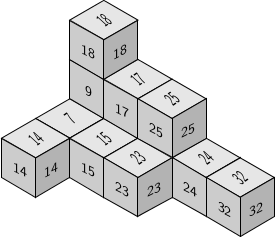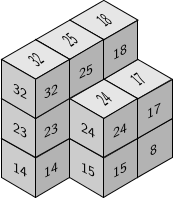# numericalsgps package for GAP

## Authors

• P. A. García-Sánchez (Universidad de Granada)

• J. J. Morais (until 2008)

0.98 dev

2006

Deposited

# Description of the package

Computing with numerical and affine semigroups

## Contents

• Definition of numerical semigroups and notable elements
• Presentations of numerical semigroups
• Constructing numerical semigroups from others
• Irreducible numerical semigroups
• Ideals of numerical semigroups
• Numerical semigroups with maximal embedding dimension
• Nonunique factorization invariatns
• Polynomials and numerical semigroups
• Affine semigroups
• Contributed functions

# Changes from last summer to current version (i)

• Fixed several small bugs
• Some deprecated functions became synonyms to maintain compatibility
• Some functions now work on numerical semigroups and ideals
• Now InfoNumSgps is used in more functions
• Added IsUniquelPresentedNumericalSemigroup, IsGenericNumericalSemigroup
• New contributions of Chris O'Neil (see the contribution appendix of the manual) for factorizations, delta sets and $$\omega$$-primality of numerical semigroups
• Added functions for semigroup of values of curves parametrized by polynomials (also local version; series)
• Numerical semigroups with a given set of pseudo-Frobenius numbers

# Changes from last summer to current version (ii)

• Added a new chapter for affine semigroups
• Added alternate catenary degrees: monotone, adjacent, equal, homogeneous
• New implementation of $$\omega$$-primality
• Added special methods for full affine semigroups (speed up factor 1000)
• Interaction with 4ti2gap, 4ti2Interface, NormalizInterface, singular and SingularInterface
• Methods implemented depending if these packages have been loaded

gap> LoadPackage("num");
#I  Please load package NormalizInterface or 4ti2Interface
#I  to have extended functionalities.
#I  Please load package SingularInterface or singular
#I  (not both) to have extended functionalities.
----------------------------------------------------------------
For help, type: ?NumericalSgps:
----------------------------------------------------------------
true
gap> NumSgpsUse
NumSgpsUse4ti2
NumSgpsUse4ti2gap
NumSgpsUseNormaliz
NumSgpsUseSingular
NumSgpsUseSingularInterface
gap> NumSgpsUse4ti2gap();
────────────────────────────────────────────────────────────────────────────────
Loading  4ti2gap 0.0.2 (GAP wraper for 4ti2)
by Pedro A. García-Sánchez (http://www.ugr.es/local/pedro) and
Alfredo Sánchez-R.-Navarro ().
Homepage: https://bitbucket.org/gap-system/4ti2gap
────────────────────────────────────────────────────────────────────────────────
true

# Unique and generic minimal presentations

For numerical semigroups

gap> s:=NumericalSemigroup(3,5,7);
<Numerical semigroup with 3 generators>
gap> IsUniquelyPresentedNumericalSemigroup(s);
true
gap> IsGenericNumericalSemigroup(s);
true
gap> MinimalPresentationOfNumericalSemigroup(s);
[ [ [ 0, 2, 0 ], [ 1, 0, 1 ] ], [ [ 3, 1, 0 ], [ 0, 0, 2 ] ],
[ [ 4, 0, 0 ], [ 0, 1, 1 ] ] ]

For affine semigroups

gap> a:=AffineSemigroup([[2,0],[0,2],[1,2],[2,1]]);
<Affine semigroup in 2 dimensional space, with 4 generators>
gap> IsUniquelyPresentedAffineSemigroup(a);
true
gap> IsGenericAffineSemigroup(a);
false
gap> MinimalPresentationOfAffineSemigroup(a);
[ [ [ 2, 0, 1, 0 ], [ 0, 2, 0, 0 ] ], [ [ 1, 0, 2, 0 ], [ 0, 0, 0, 2 ] ] ]

# Dynamic contributions by Chris O'Neil

Delta sets

gap> s:=NumericalSemigroup(43,50,72,91);
<Numerical semigroup with 4 generators>
gap> DeltaSetOfNumericalSemigroup(s);
[ 1 .. 3 ]

$$\omega$$-primality

gap> s:=NumericalSemigroup(143,150,172,191);
<Numerical semigroup with 4 generators>
gap> OmegaPrimalityOfNumericalSemigroup(s);time;
11
516
gap> a:=AsAffineSemigroup(s);
<Affine semigroup in 1 dimensional space, with 4 generators>
gap> OmegaPrimalityOfAffineSemigroup(a);time;
11
2111

Other timings

4ti2gap SingularInterface  NormalizInterface
5520  507  2111

# Another example

Now with $$S=\langle 131,301,322,311,401\rangle$$

gap> s:=NumericalSemigroup(131,301,322,311,401);
<Numerical semigroup with 5 generators>
gap> OmegaPrimalityOfNumericalSemigroup(s);time;
19
2519
gap> a:=AsAffineSemigroup(s);
<Affine semigroup in 1 dimensional space, with 5 generators>
gap> OmegaPrimalityOfAffineSemigroup(a);time;
4ti2gap  SingularInterface NormalizInterface
23554689 7196 14577957

# Semigroups of values and SABGI bases

Given a set of series (actually polynomials) $$F$$, we can compute an order bases for $$K[\![F]\!]$$, and the semigroup of values of this ring

gap> t:=X(Rationals,"t");;
gap> l:=[t^5,-t^18-t^21,t^23-t^26];;
gap> SemigroupOfValuesOfCurve_Local(l,"basis");
[ t^5, t^21+t^18, t^26, 1/2*t^42+t^39, t^47 ]
gap> MinimalGeneratingSystem(SemigroupOfValuesOfCurve_Local(l));
[ 5, 18, 26, 39, 47 ]

The same can be done for polynomials, and a SAGBI basis

gap> SemigroupOfValuesOfCurve_Global(l,"basis");
[ t^5, t^21+t^18, t^23 ]

These procedures can take advantage of 4ti2[Gap,Interface] or Singular[Interface]

# Lshapes

The factorizations Apéry sets of embedding dimension three numerical semigroups can be arranged in the space as Lshapes that teselate the plane; there are at most two of these

For embedding dimension four we were able to prove that the number of Lshapes is not bounded

gap> s:=NumericalSemigroup(7,8,9,13);;
gap> LShapesOfNumericalSemigroup(s);
[ [ [ 0, 0, 0 ], [ 1, 0, 0 ], [ 0, 1, 0 ], [ 0, 0, 1 ], [ 2, 0, 0 ],
[ 1, 1, 0 ], [ 0, 2, 0 ], [ 0, 1, 1 ], [ 0, 0, 2 ], [ 1, 2, 0 ],
[ 0, 3, 0 ], [ 0, 2, 1 ], [ 0, 4, 0 ] ],
[ [ 0, 0, 0 ], [ 1, 0, 0 ], [ 0, 1, 0 ], [ 0, 0, 1 ], [ 2, 0, 0 ],
[ 1, 1, 0 ], [ 1, 0, 1 ], [ 0, 1, 1 ], [ 0, 0, 2 ], [ 2, 0, 1 ],
[ 1, 1, 1 ], [ 1, 0, 2 ], [ 2, 0, 2 ] ] ]# Numerical semigroups with given type

Let $$S$$ be a numerical semigroup and $$h\in \mathbb Z\setminus S$$

If $$h+S\setminus\{0\}\subset S$$, then $$h$$ is a pseudo-Frobenius number of $$S$$

Their cardinality is the Cohen-Macaulay type of $$K[\![S]\!]$$

gap> NumericalSemigroupsWithPseudoFrobeniusNumbers([15,19,21]);
[ <Numerical semigroup>, <Numerical semigroup>, <Numerical semigroup>,
<Numerical semigroup> ]
gap> List(last,MinimalGeneratingSystem);
[ [ 8, 9, 14, 20 ], [ 9, 11, 13, 14, 16, 17 ], [ 10, 12, 13, 14, 16, 17, 18 ],
[ 11, 12, 13, 14, 16, 17, 18, 20 ] ]
gap> Length(NumericalSemigroupsWithFrobeniusNumber(21));
1828

# Affine semigroups

Affine semigroups can be defined in different ways

• by generators
• by equations (some in congruences): full affine semigroups
• by inequalities: normal affine semigroups

For instance the set of zero-sum sequences of $$\mathbb Z_2^2$$ can be defined as

gap> a:=AffineSemigroup("equations",[[[1,0,1],[0,1,1]],[2,2]]);
<Affine semigroup>
gap> GeneratorsOfAffineSemigroup(a);
[ [ 0, 0, 2 ], [ 0, 2, 0 ], [ 2, 0, 0 ], [ 1, 1, 1 ] ]

Or the semigroup of points of integer coordinates $$(x,y)$$ with $$2x\ge y$$ and $$3y\ge x$$ as

gap> a:=AffineSemigroup("inequalities",[[2,-1],[-1,3]]);
<Affine semigroup>
gap> GeneratorsOfAffineSemigroup(a);
[ [ 1, 1 ], [ 1, 2 ], [ 2, 1 ], [ 3, 1 ] ]

# Conclusion

New research leads to faster algorithms, and the need of faster algorithms also leads to new research

## Sources

• Our research
• Meetings (specially IMNS's)
• zbMATH reviews
• referee reports